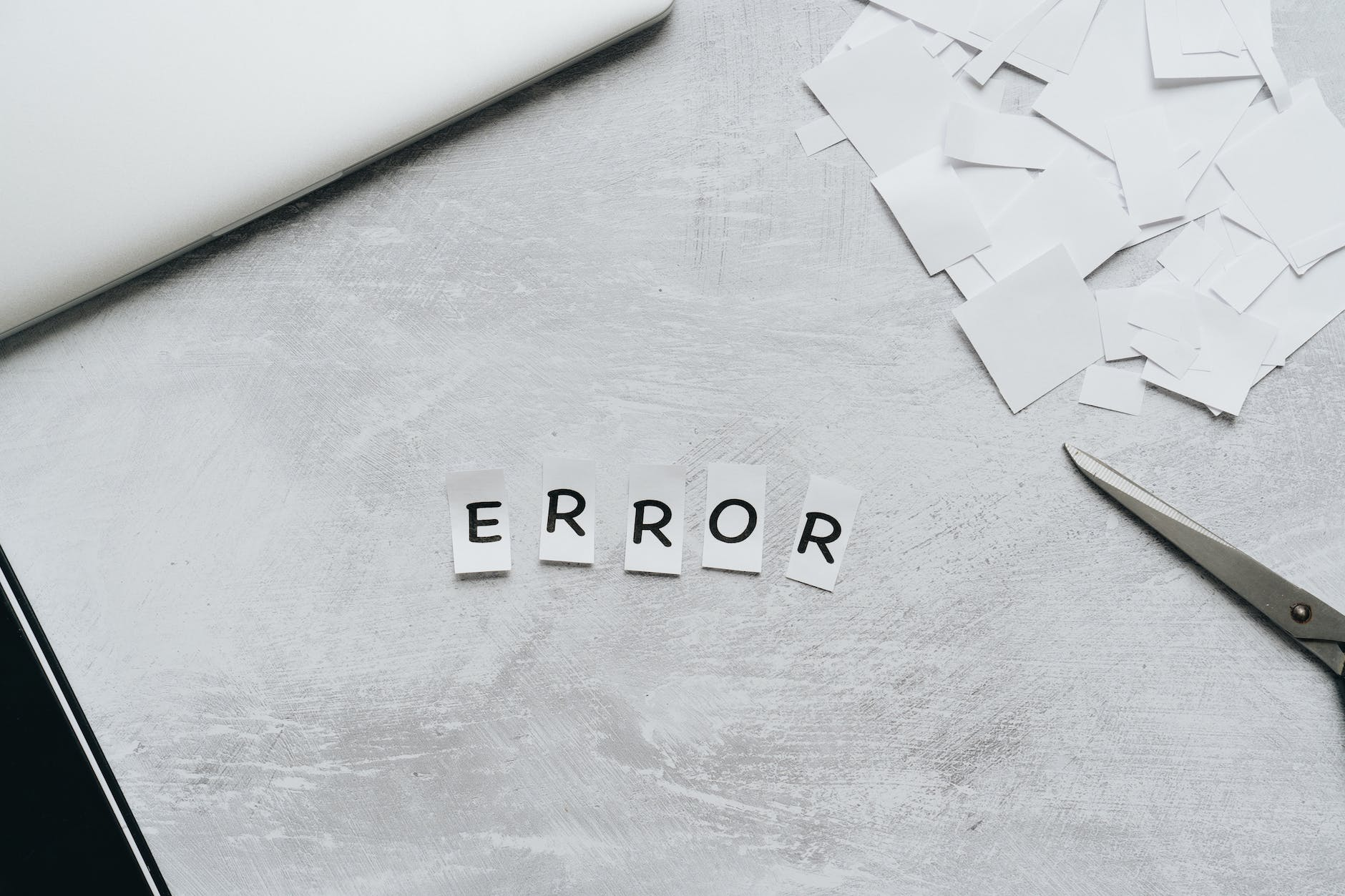NashTech Insights# Error handling in Scala###### Rakhi PareekIn Scala, error handling is typically done using exceptions and the `try-catch` construct. Here’s an overview of how error handling is done in Scala:

### Throwing Exceptions

To raise an exception, you can use the `throw` keyword followed by an instance of a subclass of `java.lang.Throwable`. For example:

``````throw new IllegalArgumentException("Invalid argument")
``````

### Handling Exceptions

To catch and handle exceptions, you can use the `try-catch` construct. The code that may potentially throw an exception is placed inside the `try` block, and one or more `catch` blocks are used to specify how to handle specific types of exceptions. For example:

``````try {
// Here we'll write code that may throw an exception
} catch {
case ex: IllegalArgumentException =>
println("Invalid argument: " + ex.getMessage)
case ex: Exception =>
println("An error occurred: " + ex.getMessage)
}
``````

In the above example, if an `IllegalArgumentException` is thrown in the `try` block, it will be caught by the first `catch` block. If any other type of exception is thrown, it will be caught by the second `catch` block. A code can have multiple `catch` blocks to handle different types of exceptions.

### Finally Block

You can also include a `finally` block after the `catch` block(s) to specify code that should be executed regardless of whether an exception was thrown or not. For example:

``````try {
// Here we'll write code that may throw an exception
} catch {
case ex: Exception =>
println("An error occurred: " + ex.getMessage)
} finally {
println("This will always execute.")
}
``````

The code inside the `finally` the block will be executed whether an exception was caught or not.

### Throwing and Catching Custom Exceptions

You can define your own exception classes by extending `java.lang.Exception` or any of its subclasses. For example:

``````class MyCustomException(message: String) extends Exception(message)

try {
// Here we'll write code that may throw an exception
throw new MyCustomException("Something went wrong")
} catch {
case ex: MyCustomException =>
println("Custom exception caught: " + ex.getMessage)
}
``````

In the above example, a custom exception `MyCustomException` is thrown and caught in the `catch` block.

### Functional Error Handling

Scala also provides functional constructs like `Try`, `Either`, and `Option` that can be used for more concise and expressive error handling. These constructs avoid the use of exceptions and provide a more functional style of error handling.

#### Try

It represents a computation that may either result in a value (`Success`) or an exception (`Failure`). It’s used for operations that may throw exceptions.

``````import scala.util.{Try, Success, Failure}

def divide(a: Int, b: Int): Try[Int] = {
Try(a / b)
}

val result1 = divide(10, 2)
result1 match {
case Success(value) => println(s"Result: \$value")
case Failure(exception) => println(s"Error: \${exception.getMessage}")
}

val result2 = divide(10, 0)
result2 match {
case Success(value) => println(s"Result: \$value")
case Failure(exception) => println(s"Error: \${exception.getMessage}")
}
``````

In the example above, we define a function called `divide` that takes two integers `a` and `b`. It attempts to divide `a` by `b` and returns a `Try[Int]` which represents either the successful result or the encountered exception.

We then invoke the `divide` function with different arguments and pattern match on the result using `match` block. If the division is successful, we print the result using `Success(value)`. If an exception occurs during division, we handle the failure case using `Failure(exception)` and print the error message using `exception.getMessage()`.

In the example, the first division (`divide(10, 2)`) is successful, so it prints “Result: 5”. The second division (`divide(10, 0)`) results in an exception (`java.lang.ArithmeticException: / by zero`), so it prints “Error: / by zero”.

#### Either

It represents a value that can be either a left (`Left`) or a right (`Right`) value. It’s often used to represent a result that can be either successful or contain an error.

``````import scala.util.{Try, Success, Failure}

object EitherExceptionHandlingExample extends App {

def divide(dividend: Int, divisor: Int): Either[String, Int] = {
if (divisor == 0) {
Left("Cannot divide by zero.")
} else {
Try(dividend / divisor) match {
case Success(result) => Right(result)
case Failure(ex) => Left(s"An exception occurred: \${ex.getMessage}")
}
}
}

val result1 = divide(10, 2)
println(result1)  // Right(5)

val result2 = divide(10, 0)
println(result2)  // Left(Cannot divide by zero.)

val result3 = divide(10, "abc".toInt) // This will throw a NumberFormatException
println(result3)  // Left(An exception occurred: For input string: "abc")
}
``````

In this example, the `divide` function takes two parameters, and returns an Either[String, Int]. If the divisor is 0, it returns a Left containing the error message “Cannot divide by zero.” Otherwise, it tries to perform the division operation using, which can potentially throw `java.lang.ArithmeticException` if the divisor is 0. If the division is successful, it returns a `Right` containing the result. If an exception occurs, it returns a `Left` containing the error message extracted from the exception.

In the `main` method, we demonstrate the usage of the `divide` function with different inputs.

#### Option

It represents an optional value that can be either `Some(value)` or `None`. It’s used when a value may or may not be present.

These functional constructs allow you to handle errors in a more type-safe and composable manner, promoting functional programming principles.

``````import scala.util.Try

def divide(a: Int, b: Int): Option[Int] = {
Try(a / b).toOption
}
val result: Option[Int] = divide(10, 2)

result match {
case Some(value) => println(s"Result: \$value")
case None => println("Division by zero!")
}
``````

In this case, if the `divide` function returns Some(value), the pattern matching will execute the first case and print the result. If it returns `None`, the second case will be executed, indicating a division by zero.

## Conclusion

This blog covers the basics of error handling in Scala using exceptions and the `try-catch` construct, as well as introducing the functional constructs for error handling. Remember that the choice of error-handling approach depends on the specific requirements of your application and the programming paradigm you prefer to follow.